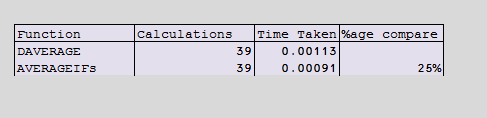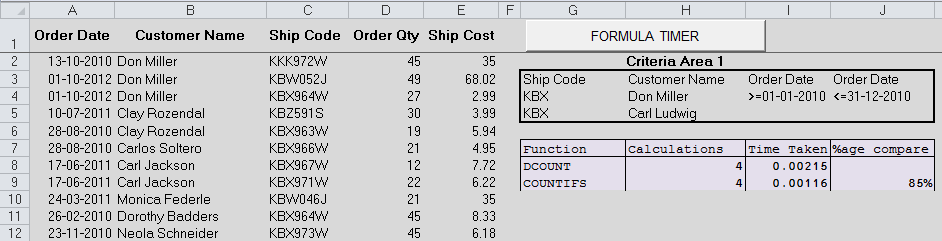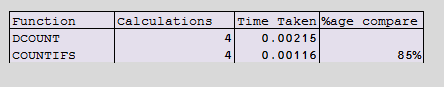# DAVERAGE vs AVERAGEIF & DCOUNT vs COUNTIF

We have been exploring on database functions for the last two articles ( EXCEL DATABASE FUNCTION and EXCEL DSUM FUNCTION.) This article will examine  DAVERAGE & DCOUNT and how they compare with their equivalent AVERAGEIFS & COUNTIFS.

Will the Database functions prevail in terms of speed over their IFS equivalent?

## DAVERAGE vs AVERAGEIFS

For example using below data compute the average ordered quantity by Don Miller for the year 2010?`=DAVERAGE(Orders,"Order Qty",G3:I4) =39`
`=AVERAGEIFS(D2:D8400,B2:B8400,G4,A2:A8400,H4,A2:A8400,I4) =39`

### RECALCULATION SPEED COMPARISON

As shown in the figure below AVERAGEIFS is a better choice (25% faster than DAVERAGE). The percentage incremental speed differs based on the complexity of the criteria but AVERAGEIFS always recalculates faster.

All the same, DAVEARAGE can handle complex criteria better than AVERAGEIFS## DCOUNT vs COUNTIFS

For example, count the orders placed by Either Don Miller OR Carl Ludwig whose ship code starts with KBX for the year 2010?`=DCOUNT(Orders,"Order Qty",G3:J5)=4`
`{=SUM(COUNTIFS(B2:B8400,H4:H5,A2:A8400,I4,A2:A8400,J4,C2:C8400,G4&"*"))}=4`

#### NB:

Since this is a COUNTIFS OR, we use an array formula as shown above.

### RECALCULATION SPEED COMPARISON

As shown in the figure below COUNTIFS is a better choice (85% faster than DCOUNT). The percentage incremental speed differs based on the complexity of the criteria but COUNTIFS always recalculates faster.

All the same, DCOUNT can handle complex criteria better than COUNTIFS## SUMMARY:

Unless you have  very complex criteria that AVERAGEIFS & COUNTIFS cannot handle, avoid DCOUNT & DAVERAGE as they will slow down your calculations.